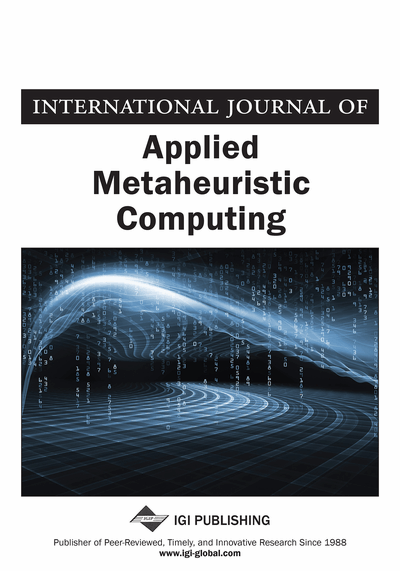# A DIMMA-Based Memetic Algorithm for 0-1 Multidimensional Knapsack Problem Using DOE Approach for Parameter Tuning

Masoud Yaghini (Iran University of Science and Technology, Iran), Mohsen Momeni (Iran University of Science and Technology, Iran) and Mohammadreza Sarmadi (Iran University of Science and Technology, Iran)
DOI: 10.4018/jamc.2012040104
Available
\$37.50
No Current Special Offers

## Abstract

Multidimensional 0-1 Knapsack Problem (MKP) is a well-known integer programming problems. The objective of MKP is to find a subset of items with maximum value satisfying the capacity constraints. A Memetic algorithm on the basis of Design and Implementation Methodology for Metaheuristic Algorithms (DIMMA) is proposed to solve MKP. DIMMA is a new methodology to develop a metaheuristic algorithm. The Memetic algorithm is categorized as metaheuristics and is a particular class of evolutionary algorithms. The parameters of the proposed algorithm are tuned by Design of Experiments (DOE) approach. DOE refers to the process of planning the experiment so that appropriate data that can be analyzed by statistical methods will be collected, resulting in valid and objective conclusions. The proposed algorithm is tested on several MKP standard instances from OR-Library. The results show the efficiency and effectiveness of the proposed algorithm.
Article Preview
Top

## 1. Introduction

The Multidimensional 0-1 Knapsack Problem (MKP) is a well-known optimization problem, which is identified to be an NP-hard problem. The mathematical formulation of MKP can be stated as follows:

(1) (2) (3) where n is a set of items with worthy profits cj ≥ 0 and m represents the dimensions of a knapsack, with capacities bi ≥ 0. Each item j consumes an amount aij ≥ 0 from each dimension i and the binary decision variables xj indicate which items are selected. The aim of this problem is to choose a subset of items with maximum total profit. For each constraint i = {1, 2, ..., m}, aij units are consumed from capacity bi, if item j is selected, where the selected items need not exceed the available capacities (Kellerer et al., 2004; Akçay et al., 2007).

The practical and theoretical importance of MKP has led to a large body of literature. Some exact, heuristic and metaheuristic methods have been suggested to solve MKPs. Fréville (2004) proposes a good review of the related problems, applications, and solution techniques.

A key step in designing an efficient exact solution method for such problem is to establish a tight upper bound on the optimal value. Quadri et al. (2007) present an effective upper bound method based on a linearization and surrogate relaxation. Boussier et al. (2010) propose a multi-level search strategy for MKP. This strategy is primarily based on the reduced costs of the non-basic variables of the LP-relaxation solution. Pi et al. (2008) use the linear relaxation solutions to generate high quality samples used for solving the local pickup and delivery problem, and the discrete facility location problem.

Several heuristic methods have been proposed to solve MKP. Akçay et al. (2007) propose a greedy heuristic which orders items by their values multiplied by the maximum number of copies of an item that could be accommodated with the resources. Puchinger et al. (2010) propose a heuristic algorithm which exploits the core problem approach proposed by Balas and Zemel (1980) for the one-dimensional knapsack problem. Their best solutions, requiring less CPU time, are dominated by those presented in Vimont et al. (2008). Hanfi and Glover (2007) offer a better exploitation of nested inequalities and surrogate constraints on MKP than Osorio et al. (2002), but they do not offer computational results. Della Croce and Grosso (2012) propose a new heuristic procedure for a parallel computing environment embedding core problem approaches and a branching scheme based on reduced costs of the corresponding LP relaxation solution value.

Fleszar and Hindi (2009) introduce several heuristics for MKP that are based on solving the LP relaxation of the problem. Hill et al. (2012) introduce problem-size reduction heuristics using the dual variables to formulate a Lagrangian relaxation of the original problem. Angelelli et al. (2010) apply the kernel search framework to solve MKP. Wilbaut et al. (2009) develop an iterative scheme which is based on a dynamic fixation of the variables.

## Complete Article List

Search this Journal:
Reset
Volume 13: 4 Issues (2022): 2 Released, 2 Forthcoming
Volume 12: 4 Issues (2021)
Volume 11: 4 Issues (2020)
Volume 10: 4 Issues (2019)
Volume 9: 4 Issues (2018)
Volume 8: 4 Issues (2017)
Volume 7: 4 Issues (2016)
Volume 6: 4 Issues (2015)
Volume 5: 4 Issues (2014)
Volume 4: 4 Issues (2013)
Volume 3: 4 Issues (2012)
Volume 2: 4 Issues (2011)
Volume 1: 4 Issues (2010)
View Complete Journal Contents Listing